## Lagrange Multiplier

Used to find the Extremum ofsubject to the constraint, where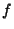and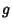are functions with continuous first Partial Derivatives on the Open Set containing the curve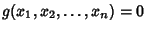, andat any point on the curve (whereis the Gradient). For an Extremum to exist,(1)

But we also have(2)

Now multiply (2) by the as yet undetermined parameterand add to (1),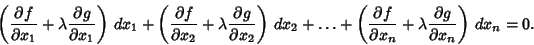(3)

Note that the differentials are all independent, so we can set any combination equal to 0, and the remainder must still give zero. This requires that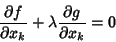(4)

for all, ...,. The constantis called the Lagrange multiplier. For multiple constraints,,, ...,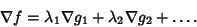(5)

Arfken, G. Lagrange Multipliers.'' §17.6 in Mathematical Methods for Physicists, 3rd ed. Orlando, FL: Academic Press, pp. 945-950, 1985.